Courses

# Analogy Questions with Answers LR Notes | EduRev

## Banking Exams : Analogy Questions with Answers LR Notes | EduRev

The document Analogy Questions with Answers LR Notes | EduRev is a part of the Banking Exams Course General Intelligence and Reasoning for SSC CGL.
All you need of Banking Exams at this link: Banking Exams

Directions to Solve
Each of the following questions consists of two sets of figures. Figures A, B, C and D constitute the Problem Set while figures 1, 2, 3, 4 and 5 constitute the Answer Set. There is a definite relationship between figures A and B. Establish a similar relationship between figures C and D by selecting a suitable figure from the Answer Set that would replace the question mark (?) in fig. (D).

Question 1)
Select a suitable figure from the Answer Figures that would replace the question mark (?).
Problem Figures: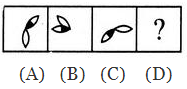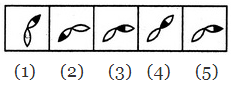A. 1
B. 2
C. 3
D. 4
E. 5
Solution:
The half-shaded leaf rotates 135ºAnti Clock Wise and the un-shaded leaf rotates 135ºClock Wise.

Question 2)
Select a suitable figure from the Answer Figures that would replace the question mark (?).
Problem Figures: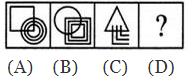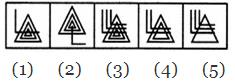A. 1
B. 2
C. 3
D. 4
E. 5
Solution:
The upper element is converted to an element similar to the lower elements and each one of the lower elements is converted to an element similar to the upper element.

Question 3)
Select a suitable figure from the Answer Figures that would replace the question mark (?).
Problem Figures: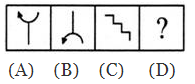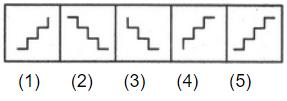A. 1
B. 2
C. 3
D. 4
E. 5
Solution: The figure gets vertically inverted.

Question 4) Select a suitable figure from the Answer Figures that would replace the question mark (?).
Problem Figures: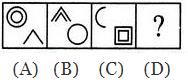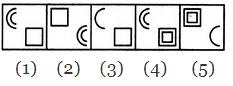Solution: Each one of the upperelementsisreplaced by an element similar to the lower element(s) and each one of the lower elements is replaced by an element similar to the upper element(s).

Question 5)
Select a suitable figure from the Answer Figures that would replace the question mark (?).
Problem Figures: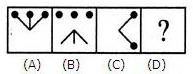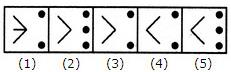Solution:
Except for the dots, the remaining part of the figure rotates through 180o and shifts to the opposite side of the square boundary.

Offer running on EduRev: Apply code STAYHOME200 to get INR 200 off on our premium plan EduRev Infinity!

## General Intelligence and Reasoning for SSC CGL

4 videos|11 docs|38 tests

,

,

,

,

,

,

,

,

,

,

,

,

,

,

,

,

,

,

,

,

,

;